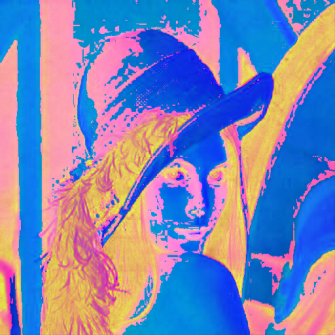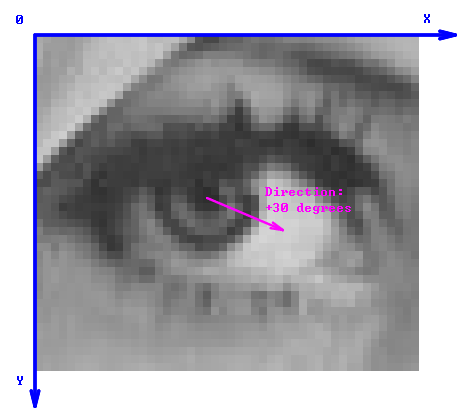# Image

### Description

The Image data type stores information about dimensions, pixel format and raw pixel values. The list of possible formats is similar to the one from the OpenCV library – there can be from 1 to 4 channels and 6 possible primitive types:

• sint8 - integer number from -128 to 127
• uint8 - integer number from 0 to 255
• sint16 - integer number from -32768 to 32767
• uint16 - integer number from 0 to 65535
• sint32 - integer number from -2147483648 to 2147483647
• real - real (floating point) number

For maximum flexibility handling of specific color spaces is left to appropriate filters.A single-channel image.A 3-channel RGB image.A 3-channel HSV image displayed as RGB.

## Geometrical Coordinates

If w and h are the image dimensions, then all real-valued coordinates within the image are included in the ranges from 0.0 to w and from 0.0 to h (right-open). The top left pixel of the image covers the square area with the top-left corner at (0.0, 0.0) and the bottom-right corner at (w, h). The X axis is directed to the right, the Y axis downwards.

Angles are in degrees – from 0 to 360. For directions, the angle 0 denotes the direction of the X axis, i.e. the direction from left to right. The angles increase in the clock-wise manner.## Memory Representation

When writing user filters you need to know the memory representation of images. The pixel data of an image is stored in a continuous memory buffer of the following structure:

• First in the buffer comes the upper-left pixel.
• Then follow the remaining pixels of the first row.
• Channels are interleaved, i.e. all components of a single pixel are located at neighboring memory addresses.
• There might be a memory padding at the end of each row (including the last one) to improve memory alignment.
• The offset of the beginning of the k'th row is equal to 'k * pitch', where 'pitch' is the byte-distance between consecutive rows. Due to row padding this might be different than 'pixel_size * width'.

## Remarks

• A region of interest, if required, is supposed to be provided as a separate object.
• Single image can allocate no more than 2GB of memory.
• Maximum image single dimension is 65535 (because regions use 16-bit integers for performance reasons).

## Library Definition

class Image
{
protected:
int                width;           // number of pixel columns
int                height;          // number of pixel rows
int                pitch;           // byte-distance between consecutive rows
PlainType::Type    type;            // type of channels
int                depth;           // number of channels
atl::Blob          blob;            // the pixel data
int                pixelSize;       // byte-size of a pixel (computed from 'type' and 'depth')

public:
/// Constructor used for images, which will be created later, e.g. by 'LoadFromBmp' function
Image();

/// Constructor used for creating new images
Image( int width, int height, PlainType::Type type, int depth, atl::Optional< const Region&amp;&> inRoi );

/// Constructor used for creating wrappers on existing data
Image( int width, int height, int pitch, PlainType::Type type, int depth, void* data );

/// Copying constructor. Performs deep data copy
Image( const Image& rhs );

~Image();

/// Deep data copy
Image& operator = ( const Image& rhs );

void                  Reset();

/// Iff the format is different then recreates the image (pixel data will be own).
/// Typically used for (re-)creating output images in image processing functions.
void                  Reset( int width, int height, PlainType::Type type, int depth,
atl::Optional< const Region&amp;&> inRoi );
void                  Reset( const Image& rhs, atl::Optional< const Region&amp;&> inRoi );

/// Turns the image into a wrapper of external data (pixel data will be NOT own)
void                  Reset( int width, int height, int pitch, PlainType::Type type, int depth,
void* data );

inline int            Width () const;
inline int            Height() const;
inline int            Pitch () const;
inline int            Area  () const;
inline Box            Frame() const;

/// Number of channels (standard RGB images have 3)
inline int            Depth() const;

/// Type of channels (standard RGB images have UINT8)
inline PlainType::Type Type()  const;

inline PixelFormat    Format() const;

/// Size of pixels in bytes
inline int            PixelSize() const;

void*                 Data();
const void*           Data() const;

/// Returns pointer to pixels memory which since that point will
/// be owned externally (this image will not delete it in the destructor).
void*                 Release();

/// Helper method for range checking
bool                  HasLocation( int x, int y ) const;
bool                  HasLocation( Location p ) const;
bool                  HasPoint( float x, float y ) const;

int                   PtrToX( const void* ptr ) const;
int                   PtrToY( const void* ptr ) const;

template < typename P&> P*          RowBegin( int i );
template < typename P&> const P*    RowBegin( int i ) const;
template < typename P&> P*          RowEnd  ( int i );
template < typename P&> const P*    RowEnd  ( int i ) const;

template < typename P&> P*          Ptr( int x, int y );
template < typename P&> const P*    Ptr( int x, int y ) const;
template < typename P&> P*          Ptr( const Location& p );
template < typename P&> const P*    Ptr( const Location& p ) const;

template < typename P&> P&          Value( int x, int y );
template < typename P&> const P&    Value( int x, int y ) const;
template < typename P&> P&          Value( const Location& p );
template < typename P&> const P&    Value( const Location& p ) const;
}


Methods:

• int Width() - returns number of pixel columns
• int Height() - returns number of pixel rows
• int Pitch() - returns byte-distance between consecutive rows
• PlainType Type() - returns type of pixel components
• int Depth() - returns number of channels
• void* Data() - returns pointer to image data buffer
• T* RowBegin<T>( int i ) - returns pointer to first element in image row
• T* RowEnd<T>( int i ) - returns pointer to first after last element in image row
• T* Ptr<T>( int x, int y ) - returns pointer to element at specified coordinates
• T& Value<T>( int i ) - allows to access pixel value at specified coordinates